# DAV Class 8 Maths Chapter 13 Worksheet 1 Solutions

The DAV Maths Book Class 8 Solutions Pdf and DAV Class 8 Maths Chapter 13 Worksheet 1 Solutions of Introduction to Graphs offer comprehensive answers to textbook questions.

## DAV Class 8 Maths Ch 13 WS 1 Solutions

Question 1.
Write the answer to each of the following questions:
(i) What is the name of the horizontal and vertical lines drawn to determine the position of any point in the Cartesian plane?
(ii) Write the name of the point where the above two lines intersect.
Solution:
(i) The horizontal line is called the x-axis or abscissa and the vertical line is called the y-axis or ordinate.
(ii) The point of intersection of two lines is called ‘origin’.Question 2.
Plot the following points on the graph:
(i) (5, 0)
(ii) (6, 2)
(iii) (0, 0)
(iv) (3, 4)
(v) (5, 6)
(vi) (1, 7)
(vii) (2, 0)
(viii) (4, 4)
Solution:
Scale:
on x-axis: 1 cm = 1 unit
on y-axis: 1 cm = 1 unit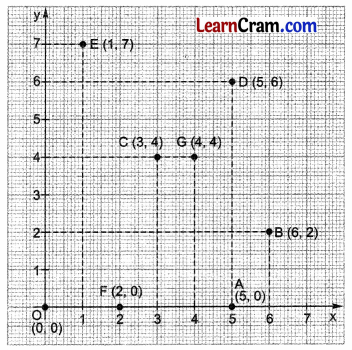Look at the given graph
(i) Point A represents (5, 0)
(ii) Point B represents (6, 2)
(iii) Point O represents (0, 0)
(iv) Point C represents (3, 4)
(v) Point D represents (5, 6)
(vi) Point E represents (1, 7)
(vii) Point F represents (2, 0)
(viii) Point G represents (4, 4)Question 3.
Find the coordinates of the points P, Q, R, and S in the following figure.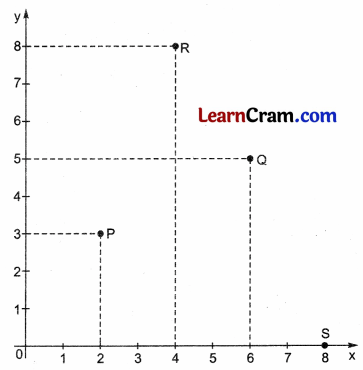Solution:
(i) The coordinates of P are (2, 3).
(ii) The coordinates of Q are (6, 5).
(iii) The coordinates of R are (4, 8).
(iv) The coordinates of S are (8, 0).

Question 4.
Plot the points A(2, 3), B(5, 3), C(5, 5) and D(2, 5) on graph. Connect the points in that order so as to get a closed figure ABCD. What type of figure do you get?
Solution:
Scale of x-axis: 1 cm = 1 unit
Scale of y-axis: 1 cm = 1 unitThe enclosed figure ABCD is a rectangle.Question 5.
Plot the points (2, 2), (4, 4), (6, 6), Join these points in pairs. Do they lie on the line passing through the origin?
Solution:
Scale on the x-axis: 1 cm = 1 unit
Scale on the y-axis: 1 cm = 1 unit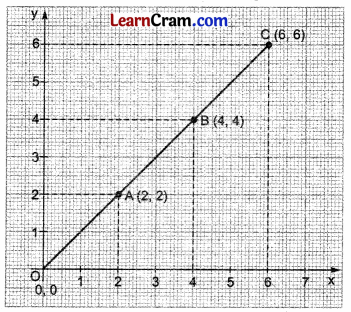Plotted points A, B, and C lie on the same line.
Yes, the line joining A, B, and C also passes through the origin.

Question 6.
(i) A point whose x-coordinate is zero, will lie on the y-axis.
(ii) A point whose y-coordinate is zero, will lie on the x-axis.
(iii) The coordinates of the origin are (1, 1).
Solution:
(i) Yes, it is true as the x-coordinate of the point is at the origin whose value is zero.
(ii) Yes, it is true as the y-coordinate of the point is at the origin whose value is zero.
(iii) False, as the coordinates of the origin are (0, 0).### DAV Class 8 Maths Chapter 13 Worksheet 1 Notes

Cartesian plane:
It is a two dimensional plane having two coordinate axes, x-axis and y-axis.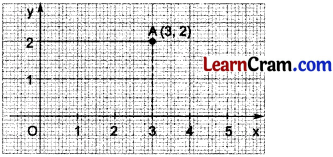• In the given figure, O is the origin, OX is x – axis and OY is y – axis.
• A is any point in the given Cartesian plane in which its x-coordinate or abscissa is 3 and the y – coordinate or ordinate is 2.
• If a point lies on x – axis, its y – coordinate is zero i.e. (x, 0) and if the point lies on y – axis, its x – coordinate is zero i.e. (0, y).
• If a point lies on the origin, its coordinates are (0, 0).

Example 1.
Plot A (4, 0), B (- 2, 3), C (0, 4), D (3, 3).
Solution:
Scale:
1 cm = 1 unit on x-axis
1 cm = 1 unit on y-axis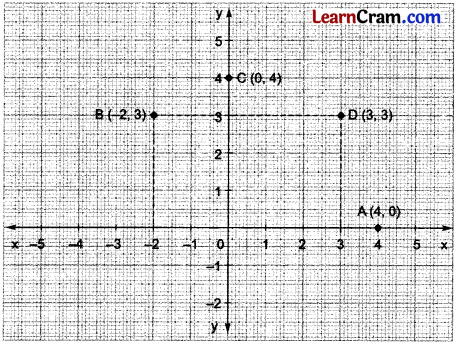Example 2.
In the given figure (see on the next page), write the following:(i) The coordinates of P.
(ii) The coordinates of Q.
(iii) Location of the point R.
(iv) The point identified by the coordinates (3, 4).
(v) The point identified by the coordinates (0, 3).
Solution:
(i) The coordinates of P are P (2, 2)
(ii) The coordinates of Q are Q (1, 4)
(iii) The coordinates of R are R (8, 1)
(iv) Point S identifies the coordinates (3, 4)
(v) Point T identifies the coordinates (0, 3).

Example 3.
Plot the points (3, 4) and (4, 3) on the same graph. What do you observe?
Solution:
The graph drawn below shows that the points A (3, 4) and B (4, 3) are different points.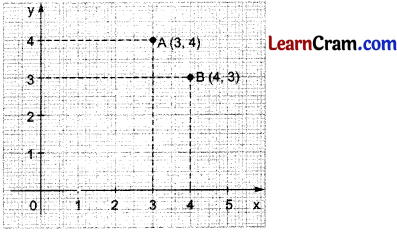Example 4.
Following table represents the attendance of the students of Viii class for a particular week.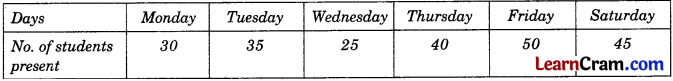Plot a graph to illustrate this information
Solution:
Scale on x-axis:
1 day = 2 cm
Scale on y-axis :
10 students = 2 cm